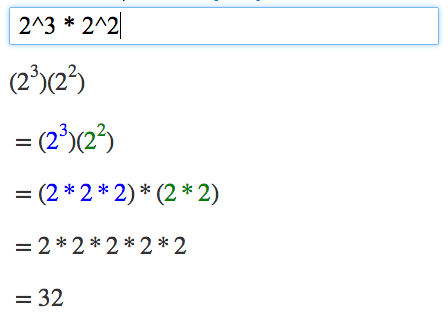# Algebra Calculator

Step-by-step calculator for algebra problems.

@
,
del
clear
=
(
)
^
÷
7
8
9
×
4
5
6
1
2
3
+
0
.
;
enter

Algebra Calculator gives step-by-step help on algebra problems.

Examples: 1+2, 1/3+1/4, 2^3 * 2^2
(x+1)(x+2) (Simplify Example), 2x^2+2y for x=5, y=3 (Evaluate Example)
y=x^2+1 (Graph Example), 4x+2=2(x+6) (Solve Example)

## How to Use the Calculator

Type your algebra problem into the text box.

For example, enter 2^3 * 2^2 into the text box to get a step-by-step explanation of how to calculate 2^3 * 2^2 = 32.### More Examples

Trying the examples on the Examples page is the quickest way to learn how to use the calculator.

### Math Symbols

If you would like to create your own math expressions, here are some symbols that the calculator understands:

- (Subtraction)
* (Multiplication)
/ (Division)
^ (Exponent: "raised to the power")
sqrt (Square Root) (Example: sqrt(9))
More Math Symbols

### Tutorial

Read the full tutorial to learn how to graph equations and check your algebra homework.

### Feedback

Please click the button at the bottom-right of the page (you should see a question-mark '?' bubble) to send your feedback. Thanks!

Learn algebra from the basics! Try the MathPapa Basic Algebra interactive lesson

Share URL: